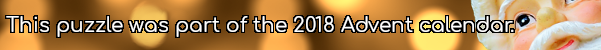mscroggs.co.uk
mscroggs.co.uksubscribeFor $$x$$ and $$y$$ between 1 and 9 (including 1 and 9), I write a number at the co-ordinate $$(x,y)$$: if $$x\lt y$$, I write $$x$$; if not, I write $$y$$.